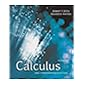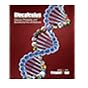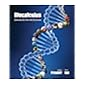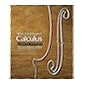# DeKUT Library

Normal view MARC view ISBD view

# Biocalculus : calculus for the life sciences / James Stewart, McMaster University and University of Toronto, Troy Day, Queen's University.

Material type:BookPublisher: Boston, MA, USA : Cengage Learning, Copyright date: ©2015Description: xlvii, 802 pages : illustrations (some color) ; 26 cm.ISBN: 1133109632; 9781133109631.Online resources: Contributor biographical information
Contents:
Functions and sequences -- Limits -- Derivatives -- Applications of derivatives -- Integrals -- Applications and integrals -- Differential equations -- Vectors and matrix models -- Multivariable calculus -- Systems of linear differential equations -- Descriptive statistitics -- Probability -- Inferential statistics.
Summary: The chief goal in Biocalculus: calculus for the life sciences is to show students how calculus relates to biology ... Although the focus is on the interface between mathematics and the life sciences, the logical structure of the book is motivated by the mathematical material. Students will come away with a sound knowledge of mathematics and an understanding of the importance of mathematical arguments. Equally important, they will also come away with a clear understanding of how these mathematical concepts and techniques are central in the life sciences.-- Provided by publishers.
Tags from this library: No tags from this library for this title.
average rating: 0.0 (0 votes)
Item type Current location Home library Call number Status Date due BarcodeBooks
General Stacks
QA 303.2 .S7 (Browse shelf) Available 2020/53981
##### Browsing DeKUT Main Campus Library Shelves , Shelving location: General Stacks Close shelf browserQA 303.2 .S65 2007 Calculas : QA 303.2 .S65 2007 Calculas : QA 303.2 .S7 Biocalculus : QA 303.2 .S7 Biocalculus : QA 303.2 .S7 1999 Multivariable calculus / QA 303.2 .S7 1999 Multivariable calculus / QA 303.2 .S7 1999 Multivariable calculus /

Includes bibliographical references and indexes.

Functions and sequences -- Limits -- Derivatives -- Applications of derivatives -- Integrals -- Applications and integrals -- Differential equations -- Vectors and matrix models -- Multivariable calculus -- Systems of linear differential equations -- Descriptive statistitics -- Probability -- Inferential statistics.

The chief goal in Biocalculus: calculus for the life sciences is to show students how calculus relates to biology ... Although the focus is on the interface between mathematics and the life sciences, the logical structure of the book is motivated by the mathematical material. Students will come away with a sound knowledge of mathematics and an understanding of the importance of mathematical arguments. Equally important, they will also come away with a clear understanding of how these mathematical concepts and techniques are central in the life sciences.-- Provided by publishers.

There are no comments for this item.